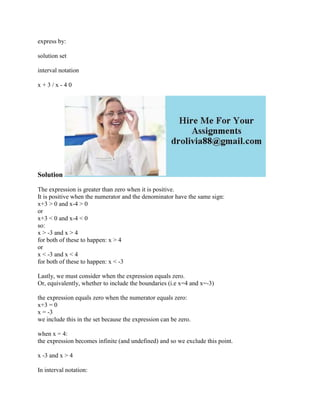Ce diaporama a bien été signalé.
Le téléchargement de votre SlideShare est en cours. ×

# express by- solution set interval notation x + 3 - x - 4 0SolutionThe.docx

Publicité
Publicité
Publicité
Publicité
Publicité
Publicité
Publicité
Publicité
Publicité
Publicité
PublicitéChargement dans…3
×

## Consultez-les par la suite

1 sur 2 Publicité

# express by- solution set interval notation x + 3 - x - 4 0SolutionThe.docx

express by:
solution set
interval notation
x + 3 / x - 4 0
Solution
The expression is greater than zero when it is positive.
It is positive when the numerator and the denominator have the same sign:
x+3 > 0 and x-4 > 0
or
x+3 < 0 and x-4 < 0
so:
x > -3 and x > 4
for both of these to happen: x > 4
or
x < -3 and x < 4
for both of these to happen: x < -3

Lastly, we must consider when the expression equals zero.
Or, equivalently, whether to include the boundaries (i.e x=4 and x=-3)

the expression equals zero when the numerator equals zero:
x+3 = 0
x = -3
we include this in the set because the expression can be zero.

when x = 4:
the expression becomes infinite (and undefined) and so we exclude this point.

x -3 and x > 4
In interval notation:
(-,-3] U (4,)
.

express by:
solution set
interval notation
x + 3 / x - 4 0
Solution
The expression is greater than zero when it is positive.
It is positive when the numerator and the denominator have the same sign:
x+3 > 0 and x-4 > 0
or
x+3 < 0 and x-4 < 0
so:
x > -3 and x > 4
for both of these to happen: x > 4
or
x < -3 and x < 4
for both of these to happen: x < -3

Lastly, we must consider when the expression equals zero.
Or, equivalently, whether to include the boundaries (i.e x=4 and x=-3)

the expression equals zero when the numerator equals zero:
x+3 = 0
x = -3
we include this in the set because the expression can be zero.

when x = 4:
the expression becomes infinite (and undefined) and so we exclude this point.

x -3 and x > 4
In interval notation:
(-,-3] U (4,)
.

Publicité
Publicité

### express by- solution set interval notation x + 3 - x - 4 0SolutionThe.docx

1. 1. express by: solution set interval notation x + 3 / x - 4 0 Solution The expression is greater than zero when it is positive. It is positive when the numerator and the denominator have the same sign: x+3 > 0 and x-4 > 0 or x+3 < 0 and x-4 < 0 so: x > -3 and x > 4 for both of these to happen: x > 4 or x < -3 and x < 4 for both of these to happen: x < -3 Lastly, we must consider when the expression equals zero. Or, equivalently, whether to include the boundaries (i.e x=4 and x=-3) the expression equals zero when the numerator equals zero: x+3 = 0 x = -3 we include this in the set because the expression can be zero. when x = 4: the expression becomes infinite (and undefined) and so we exclude this point. x -3 and x > 4 In interval notation:
2. 2. (-,-3] U (4,)